# High School Math : How to find the equation of a perpendicular line

## Example Questions

### Example Question #1 : How To Find The Equation Of A Perpendicular Line

Write an equation in slope-intercept form for the line that passes through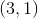and that is perpendicular to a line which passes through the two pointsand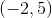.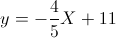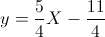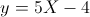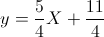Explanation:

Find the slope of the line through the two points. It is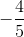.

Since the slope of a perpendicular line is the negative reciprocal of the original line, the new line's slope is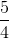. Plug the slope and one of the points into the point-slope formula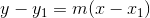. Isolate for### Example Question #2 : How To Find The Equation Of A Perpendicular Line

Find the equation of a line perpendicular to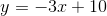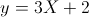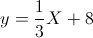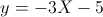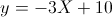Explanation:

Since a perpendicular line has a slope that is the negative reciprocal of the original line, the new slope is. There is only one answer with the correct slope.

### Example Question #3 : How To Find The Equation Of A Perpendicular Line

Find the equation (in slope-intercept form) of a line perpendicular to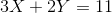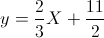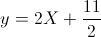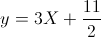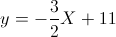Explanation:

First, find the slope of the original line, which is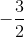. You can do this by isolating forso that the equation is in slope-intercept form. Once you find the slope, just replace thein the original equation withe the negative reciprocal (perpendicular lines have a negative reciprocal slope for each other). Thus, your answer is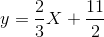### Example Question #4 : How To Find The Equation Of A Perpendicular Line

Given the equation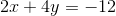and the point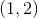, find the equation of a line that is perpendicular to the original line and passes through the given point.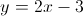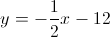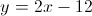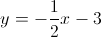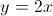Explanation:

In order for two lines to be perpendicular, their slopes must be opposites and recipricals of each other. The first step is to find the slope of the given equation: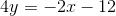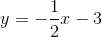Therefore, the slope of the perpendicular line must be. Using the point-slope formula, we can find the equation of the new line: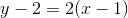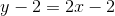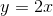### Example Question #5 : How To Find The Equation Of A Perpendicular Line

What line is perpendicular to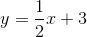through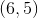?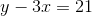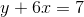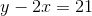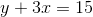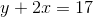Explanation:

Perdendicular lines have slopes that are opposite reciprocals.  The slope of the old line is, so the new slope is.

Plug the new slope and the given point into the slope intercept equation to calculate the intercept:or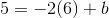, so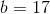.

Thus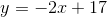, or.

### Example Question #6 : How To Find The Equation Of A Perpendicular Line

What is the equation, in slope-intercept form, of the perpendicular bisector of the line segment that connects the points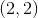and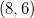?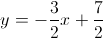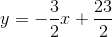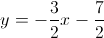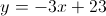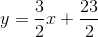Explanation:

First, calculate the slope of the line segment between the given points.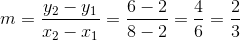We want a line that is perpendicular to this segment and passes through its midpoint. The slope of a perpendicular line is the negative inverse. The slope of the perpendicular bisector will be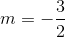.

Next, we need to find the midpoint of the segment, using the midpoint formula.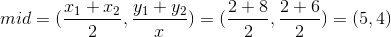Using the midpoint and the slope, we can solve for the value of the y-intercept.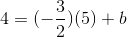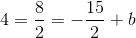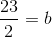Using this value, we can write the equation for the perpendicular bisector in slope-intercept form.### Example Question #7 : How To Find The Equation Of A Perpendicular Line

What line is perpendicular to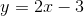through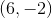?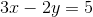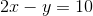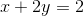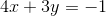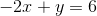Explanation:

The equation is given in the slope-intercept form, so we know the slope is.  To have perpendicular lines, the new slope must be the opposite reciprocal of the old slope, or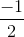Then plug the new slope and the point into the slope-intercept form of the equation: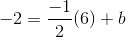so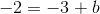so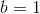So the new equation becomes: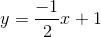and in standard form